# Millimeter Wave Phased Array Design for 5G Access Terminals – 28 GHz Band

by Hany A. Raouf, PhD          July 2020

## 1. Introduction

Although the Federal Communication Commission (FCC) has specified the frequency band (27.5 to 28.35 GHz) for 5G and the Millimeter Wave (mmWave) communication systems attracted much interests for the high data rates requirements of 5G, the mmWaves suffer from extreme free-space loss and blockage.  The communication between the base station (BS) and the access point (AP) are affected by the surrounding obstacles.  In addition, the raindrop dimensions are comparable  to the wavelength in this frequency band which produce strong electromagnetic scattering.

For the above reasons, the antenna design for mmWave communication must overcome the strong attenuation and path loss between the transmitters and the receivers. The access link must use directional high gain antennas to overcome the bath loss and satisfy the required  high data rates and user mobility.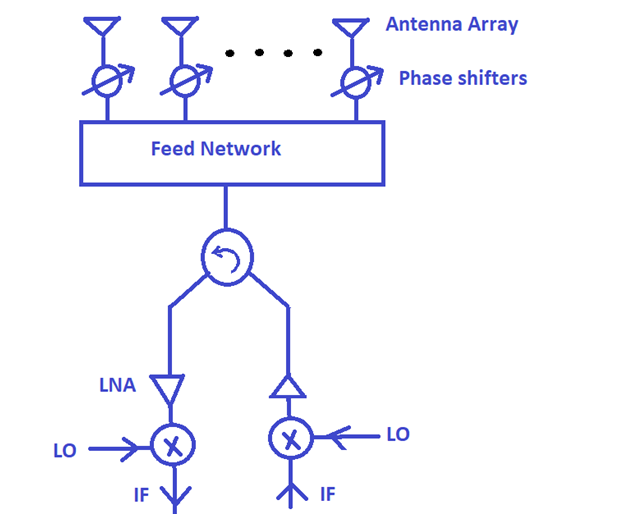Figure 1:  Block diagram for the phased array antenna system.

Figure 1 shows a block diagram for the phased array antenna system. The design of the mmWave phased arrays includes the design of the antenna array, the phase shifters, the feed network, the amplifiers, and the mixers.  The beamforming techniques were discussed in our previous blog. In this article we will focus on the design of the mmWave antenna array for access point.

## 2. Single Patch Design for 28 GHz

In the following  we will discuss the design of mmWave array of patches at 28 GHz . Figure 2(a) shows the single patch element with coaxial feeding line. The substrate material is RT-duroid 5880 with 2.33 dielectric constant.

Figure 2: Single Patch with coaxial feed line.

The S11 is simulated, using CST– Frequency domain solver, for the single patch antenna of Figure2  and the result is shown in Figure 2(b).  The result shows S11 = -34 dB at the  resonant frequency f0= 28.041 GHz with bandwidth 325 MHz.  The design of this antenna can be modified to increase the bandwidth of this antenna to 1.3 GHz as shown by the end of this article. The 3D radiation pattern of this  single element  patch antenna  is shown in Figure 3.

Figure 3:  3D directivity of the single patch antenna of Figure2.

## 3. Phased Array

Now we will use the above patch antenna to design different phased arrays for 5G access terminals at 28 GHz band. Our code takes the shape of the radiation pattern or the beamform requirements as input and calculate the array parameters, e.g. spaces between the elements and amplitudes  as outputs.

In Figure 4 we present a design of 8 elements linear array with spacing between the elements d=6.84 mm. We designed non-uniform amplitude to reduce the SLL < -30 dB .  We simulated the whole array, using CST, to consider the coupling effect between the elements of the array. The results of the directivity in the XZ-plane for scanning angle 0 deg are shown in Figures 4(a).  The results show directivity of 14 dBi, main lobe ,  half power beamwidth (HPBW) of 14 deg , and side lobe level of -32 dB. In Figure 4(b) we calculated  the directivity for scan angle 23 deg using the pattern multiplication of the single element x the array factor, which doesn’t include the coupling effect between the elements. Figure 4(b) shows the directivity of the above array in the XZ-plane.  In Figures 4(c) and (d), we simulated the whole array, using CST . The results of the directivity in the XZ-plane for scann angle 23 deg and the 3D pattern are shown in Figures 4(c) and (d) respectively.  The results of the pattern multiplication show directivity of 13.4 dBi, main lobe direction at θ=23o,  half power beamwidth (HPBW) of 15.2 , and side lobe level of -9.1 dB while the results of the actual simulation shows directivity of 13.7 dBi, main lobe direction at θ=22o,  HPBW of 15o , and side lobe level of -11.6 dB. As the scan angle increases the cross section area of the array decreases which leads to less power to the main lobe and less directivity. We can improve  this design for wide scan angles using different array shapes and amplitude distributions.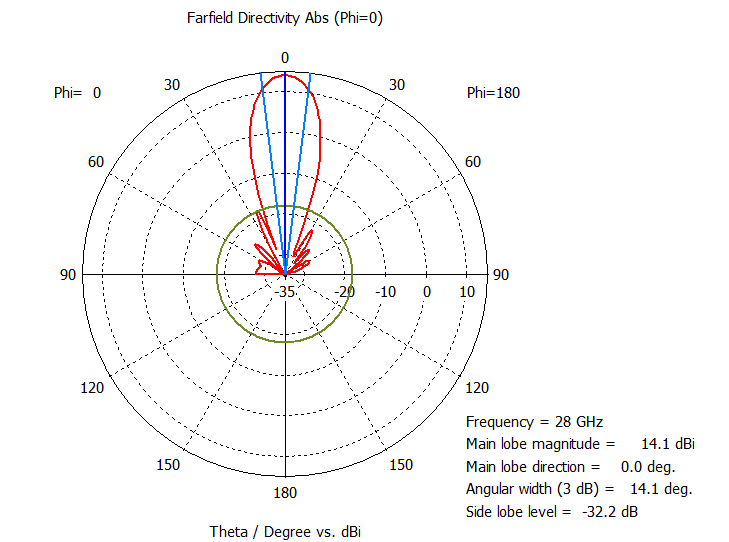(a) 8 elements linear array with non-uniform amplitude using the actual simulation of the whole array , for scan angle 0 deg.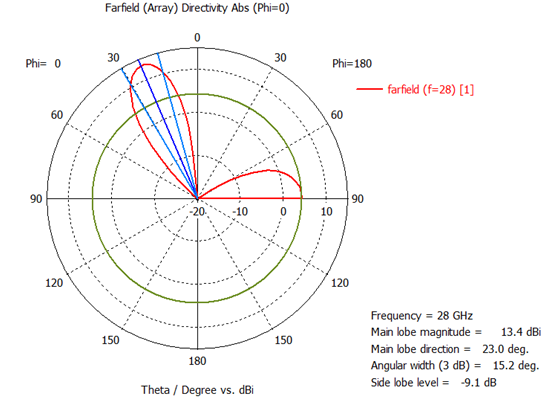(b) 8 elements linear array with non-uniform amplitude for scan angle 23 deg. The results are calculated using the pattern multiplication.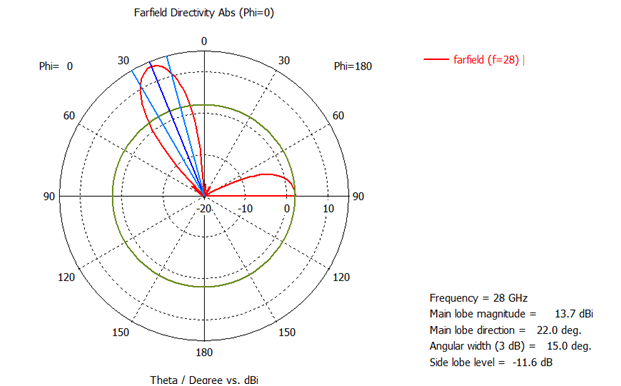(c) 8 elements linear array with non-uniform amplitude using the actual simulation of the whole array.

Figure 4: Directivity of a linear non-uniform amplitude array of 8 elements at f=28 GHz.

Figures 5 and 6 show the design of 8 x 8 rectangular array with uniform amplitude and spacing between the elements d = λ/2 = 5.36 mm. Figure 5(b) shows the directivity of the above array in the YZ-plane. The results show directivity of  23.1 dBi, half power beamwidth (HPBW) of 12.6 deg, and side lobe level of -13.4 dB  Figure 6 shows animation for the 3D directivity scanning.  The 8 x 8 rectangular array gives more than 9 dB increase in the directivity compared to the 8 elements linear array . Figure 8 shows animation for the 3D pattern scanning.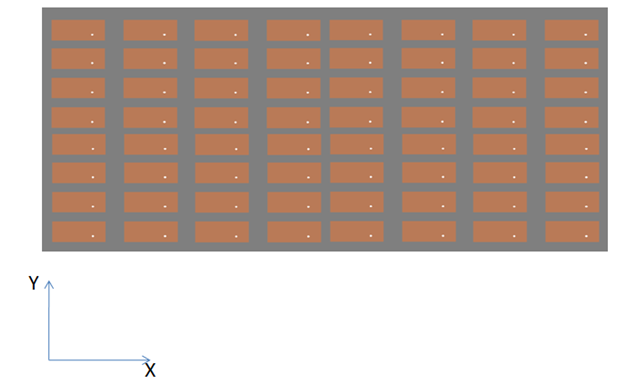(a) 8 x 8 rectangular array with spacing between the elements d = λ/2 = 5.36 mm.

Figure 5:    8 x 8 rectangular array with d = λ/2 =5.36 mm.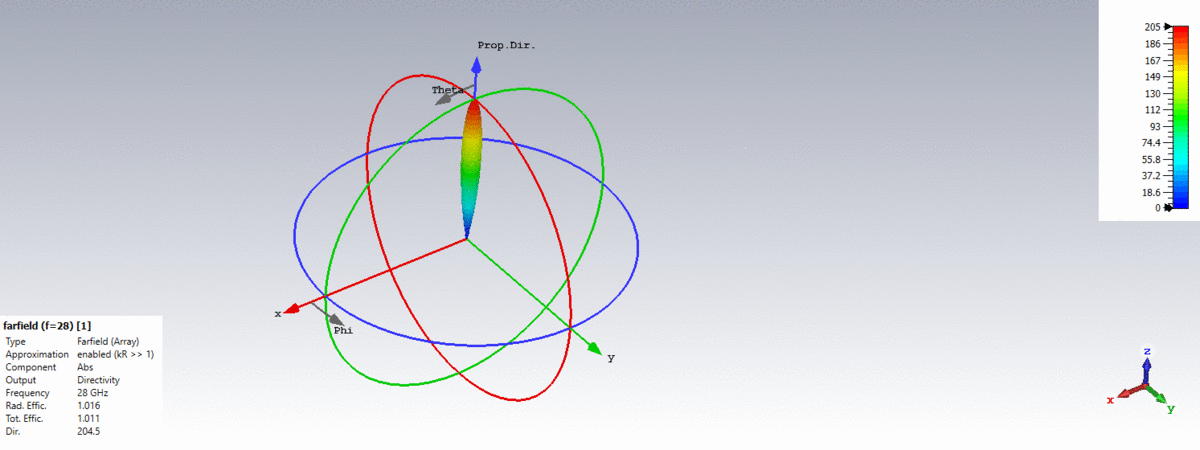Figure 6: Animation for the 3D directivity scanning of the 8 x 8 rectangular array of Figure 5.

Figures 7 and 8 show the results for  16 x 4 rectangular array with uniform amplitude and spacing between the elements d = λ/2 = 5.36 mm. Figure 7 shows the directivity of the above array in the XZ-plane. Obviously this array gives narrower beamwidth  in the XZ-plane, as shown from Figure 7, due to increasing the size of the array in the XZ-plane.  Figure 8 shows animation for the 3D pattern scanning.

Figure 7:    16 x 4 rectangular array with d = λ/2.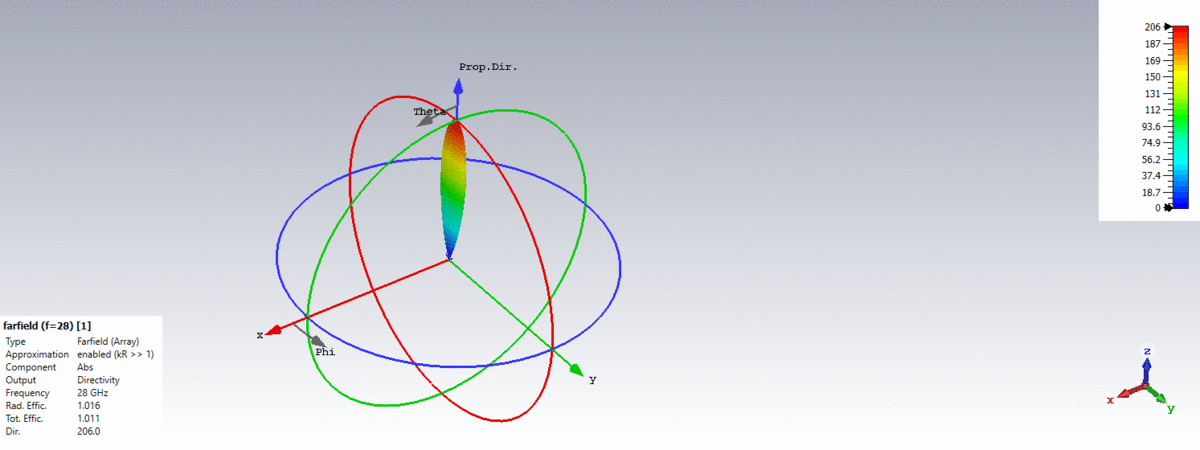Figure 8: Animation for the 3D directivity scanning of the 16 x 4 rectangular array of Figure 7.

## 4. Improving the bandwidth of the mmWave antenna  of   Figure 2

In order to improve the bandwidth of the mmWave patch antenna of Figure 2, we used partial ground plane for this antenna, as we presented in  and . The S11 is shown in Figure 9 which shows a bandwidth of 1.3 GHz for S11 < -10 dB. The details of this design will be presented in a new article.

Figure 9: S11 (dB) for the patch of Figure 2 with partial ground plane.